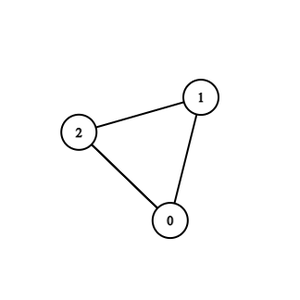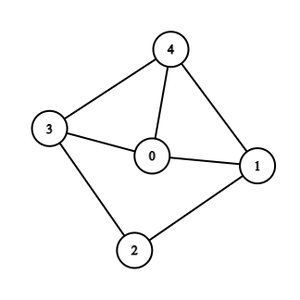# Find edges removing which does not disconnect the Graph

• Last Updated : 21 Jun, 2022

Given N vertices numbered from 0 to N – 1 and E edges to form an undirected graph. All the edges should be added in the given order. The task is to find the edges removing which do not disconnect the graph. If there are multiple possible edges return the ones which occur later in the sequence.

Examples:

Input: N = 3, E = 3, edges= {{0, 1}, {1, 2}, {0, 2}}Output: 0 2
Explanation:  Removing any one of the edges keep the graph connected.
But (0, 2) comes later in the sequence.

Input: N = 5, E = 7, edges = {{0, 1}, {1, 2}, {2, 3}, {4, 3}, {0, 4}, {4, 1}, {3, 0}}Output:
0 4
4 1
3 0
Explanation: After removing the edges (0, 4), (4, 1), (3, 0) the graph will still be connected. Hence, these three edges are extra edges.

Approach: This problem can be solved using the concept of disjoint set data structure based on the following idea:

Keep connecting the edges to each other if they are previously not been connected, if they are already connected then the current edge for sure be an extra edge.

Use disjoint sets to find if the nodes are previously connected or not. If they are connected then connect the edge and union those two sets.

Follow the below steps to solve the problem:

• Create a parent[] array to store the parent nodes of each node.
• Create a set of pairs (say ans), to store the answer.
• Run a loop through the given edges.
• Check if the vertices of the current edge are already connected or not.
• If they are connected then store this edge into the ans.
• Else, connect these two vertices and unite those two disjoint sets (i.e., make their parent same).
• Return and print ans.

Below is the implementation of the above approach:

## C++

 `// C++ code to implement the above approach`   `#include ` `using` `namespace` `std;`   `// The required Comparator Function.` `int` `find(``int` `x, vector<``int``>& parent)` `{` `    ``if` `(x == parent[x]) {` `        ``return` `x;` `    ``}` `    ``return` `parent[x]` `           ``= find(parent[x], parent);` `}`   `// Function to find extra edges` `set > extraEdges(``int` `n, ``int` `e,` `                                ``vector >& edges)` `{` `    ``set > ans;` `    ``vector<``int``> parent(n);` `    ``for` `(``int` `i = 0; i < n; i++) {` `        ``parent[i] = i;` `    ``}`   `    ``// Loop through the edges` `    ``for` `(``auto` `x : edges) {` `        ``int` `vParent = find(x, parent);` `        ``int` `uParent = find(x, parent);` `        ``if` `(vParent == uParent) {` `            ``pair<``int``, ``int``> p;` `            ``if` `(x < x) {` `                ``p = { x, x };` `            ``}` `            ``else` `{` `                ``p = { x, x };` `            ``}` `            ``ans.insert(p);` `        ``}` `        ``else` `{` `            ``parent[vParent] = uParent;` `        ``}` `    ``}`   `    ``// Return the edges` `    ``return` `ans;` `}`   `// Driver code` `int` `main()` `{` `    ``int` `N = 3, E = 3;` `    ``vector > edges` `        ``= { { 0, 1 }, { 1, 2 }, { 2, 0 }, { 0, 2 } };` `    ``set > ans = extraEdges(N,` `                                          ``E, edges);` `    ``for` `(``auto``& edge : ans) {` `        ``cout << edge.first << ``" "` `<< edge.second << ``"\n"``;` `    ``}` `    ``return` `0;` `}`

## C#

 `// C# program to implement above approach` `using` `System;` `using` `System.Collections;` `using` `System.Collections.Generic;`   `class` `GFG` `{`   `    ``// The required Comparator Function. ` `    ``static` `int` `find(``int` `x, List<``int``> parent) ` `    ``{ ` `        ``if` `(x == parent[x]) { ` `            ``return` `x; ` `        ``} ` `        ``return` `parent[x] = find(parent[x], parent); ` `    ``} `   `    ``// Function to find extra edges ` `    ``static` `SortedSet extraEdges(``int` `n, ``int` `e, List> edges) ` `    ``{ ` `        ``SortedSet ans = ``new` `SortedSet(``new` `Comp()); ` `        ``List<``int``> parent = ``new` `List<``int``>(); ` `        ``for` `(``int` `i = 0; i < n; i++) { ` `            ``parent.Add(i);` `        ``} `   `        ``// Loop through the edges ` `        ``foreach` `(List<``int``> x ``in` `edges) { ` `            ``int` `vParent = find(x, parent); ` `            ``int` `uParent = find(x, parent); ` `            ``if` `(vParent == uParent) { ` `                ``pair p = ``new` `pair(0, 0); ` `                ``if` `(x < x) { ` `                    ``p = ``new` `pair(x, x); ` `                ``} ` `                ``else` `{ ` `                    ``p = ``new` `pair(x, x); ` `                ``} ` `                ``ans.Add(p); ` `            ``} ` `            ``else` `{ ` `                ``parent[vParent] = uParent; ` `            ``} ` `        ``} `   `        ``// Return the edges ` `        ``return` `ans; ` `    ``}`   `    ``// Driver Code` `    ``public` `static` `void` `Main(``string``[] args){` `        `  `        ``int` `N = 3, E = 3; ` `        ``List> edges = ``new` `List>{` `            ``new` `List<``int``>{ 0, 1 },` `            ``new` `List<``int``>{ 1, 2 },` `            ``new` `List<``int``>{ 2, 0 },` `            ``new` `List<``int``>{ 0, 2 }` `        ``}; ` `        ``SortedSet ans = extraEdges(N,  E, edges); ` `        ``foreach` `(pair edge ``in` `ans) {` `            ``Console.WriteLine(edge.first + ``" "` `+ edge.second);` `        ``}`   `    ``}` `}`   `public` `class` `pair{` `    ``public` `int` `first;` `    ``public` `int`  `second;` `    ``public` `pair(``int` `first,``int` `second){` `        ``this``.first = first;` `        ``this``.second = second;` `    ``}` `}`   `class` `Comp : IComparer{` `    ``public` `int` `Compare(pair o2,pair o1){` `        ``if``(o1.first == o2.first){` `            ``return` `o1.second - o2.second;` `        ``}` `        ``return` `o1.first - o2.first;` `    ``}` `}`

## Python3

 `# Python3 code to implement the above approach`   `def` `find(x,parent):`   `    ``if` `(x ``=``=` `parent[x]):` `        ``return` `x` `    `  `    ``parent[x] ``=` `find(parent[x], parent)` `    ``return` `parent[x]`   `# Function to find extra edges` `def` `extraEdges(n,e,edges):`   `    ``ans ``=` `set``()` `    ``parent ``=` `[i ``for` `i ``in` `range``(n)]`   `    ``# Loop through the edges` `    ``for` `x ``in` `edges:` `        ``vParent ``=` `find(x[``0``], parent)` `        ``uParent ``=` `find(x[``1``], parent)` `        ``if` `(vParent ``=``=` `uParent):` `            ``p ``=` `()` `            ``if` `(x[``0``] < x[``1``]):` `                ``p ``=` `( x[``0``], x[``1``] )` `            `  `            ``else``:` `                ``p ``=` `( x[``1``], x[``0``] )` `        `  `            ``ans.add(p)` `        ``else``:` `            ``parent[vParent] ``=` `uParent` `        `    `    ``# Return the edges` `    ``return` `ans`   `# Driver code`   `N,E ``=` `3``,``3` `edges ``=` `[ [ ``0``, ``1` `], [ ``1``, ``2` `], [ ``2``, ``0` `], [ ``0``, ``2` `] ]` `ans ``=` `extraEdges(N, E, edges)` `for` `edge ``in` `ans:` `    ``print``(f``"{edge} {edge}"``)`   `# This code is contributed by shinjanpatra`

Output

`0 2`

Time Complexity: O(E * log(N))
Auxiliary Space: O(N)

My Personal Notes arrow_drop_up
Recommended Articles
Page :